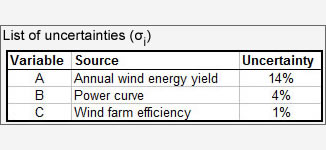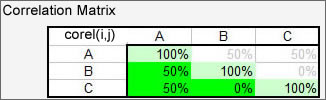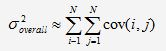Finance » Financial Modelling

# Estimating the uncertainty of revenues and investment decisions

Clean energy projects tend to require a large capital outlay upfront. With low ongoing operating costs, the capacity to pay back the capital is largely dependent on the revenue stream. Unlike costs, however, revenues are subject to variations from the model forecast beyond the control of management. Reasons for this include market price movements and variations in the annual energy production.

Annual Revenues = Annual Energy Production (AEP) x Unit Price

Price Movements: We assume here that the unit price is known, as is often the case in renewable energy projects, either because of guaranteed subsidy payments or a power purchase agreement. The technique described below can not be applied to price uncertainty. There are other techniques to mitigate energy price risks.

Annual Energy Production (AEP): The AEP itself depends on multiple factors, such as the annual incoming energy (e.g. annual irradiance), an average conversion factor for a single device and an efficiency factor in case multiple devices are used in tandem (e.g. wind farm or solar farm):

## Uncertainty of Annual Energy Production

As the price is either fixed or market risk otherwise mitigated, the focus of investors and bankers is on the uncertainty of the estimate for the annual energy production. To determine the probability of reaching higher or lower values than the base case, or conversely to determine the AEP that will be reached with more or less probability than 50%, the following algorithm can be used:1. Identify sources of uncertainty within the base-case calculation
2. Estimate uncertainties (or variances) of those sources
3. Determine if any of the sources are correlated.
4. Calculate the overall uncertainty.

### 1. Identify sources of uncertainty

Determine which parameters in the calculation for the AEP are uncertain due to
• natural fluctuations: This applies to any renewable source - for instance, annual wind speed or annual irradiation.
• manufacturing tolerances: The power curve of any wind turbine is slightly different from the data sheet whilst power output of a pv module can vary +/-5%.
• model simplifications: For instance, the effect of reduced efficiency by using multiple wind turbines in a wind farm is reflected in one single number: wind farm efficiency. Due to the complexity of the underlying processes, this number can only ever be a guess!

### 2. Estimate variancesIn the second step, quantify the expected (or suspected) variances in those parameters in terms of standard deviation σ. The values for those can be sourced from:
• Measurements: For instance, taken from wind atlas or own wind measurement campaign. The variance should be adjusted upwards if measurements have not been taken at the exact site or only over a short period of time.
• Data sheets: Figures provided by manufacturers for power curves.
• Experience

### 3. Determine correlationsCorrelation measures the degree of relationship between two random processes. A correlation coefficient corel(A,B) expresses the impact of a change in parameter A on parameter B. Values range from zero (uncorrelated) to +/-100%. The correlation matrix takes into account all combinations of parameters. Note that the coefficients on the main diagonal are 100%, and that corel(A,B) = corel(B,A). Again, sources for values include actual measurements, experience and just rough guesses.

### 4. Calculate overall uncertaintyIn the final step, the overall uncertainty can be calculated from the elements of the covariance matrix whose elements can be calculated from the coefficients of the correlation matrix and the uncertainties of the parameters. The overall expected variance is then:which is the sum of all the elements in the covariance matrix.

## Risk assessment using exceedance probabilities

We are assuming that values for the annual energy production fall into a normal distribution centered around the expected forecast (from the base case) and a variance as calculated above.This assumption is ok in most instances as long as the standard deviations of the individual parameters are well below 100%. The normal distribution provides the cumulative probability that the forecast average level is exceeded (exceedence probability).

### P50, P75, P95

A "PXX" denotes the annual energy production level that is reached with a probability of XX%. Hence, P95 means: There is a chance of 5% that the P95 level will not be reached. P50 of course is the base case, as there is a 50:50 chance it will be exceeded.

For instance, if the base-case AEP is 200kWh and overall uncertainty is σ=9%, then the P95 is at 173kWh while the P75 is at 192kWh. With more uncertainty, those values decrease!

The PXX values are widely used by potential investors and banks as basis for financing decisions.

Typically, banks will apply the P90 or P95 level for their revenue forecast in order to determine if the interest cover is sufficient. Equity investors on the other hand may use the P75 or even P50 levels. However, as exceedence probabilities go lower, yield expectations of the investors will increase. While banks may lend money based on P95 level at 5%, an investor that bases their financing decision on the P50 case will expect more like 15%.

More uncertainty results in lower PXX levels and therefore higher cost of capital, as investors have to asssume more risk.